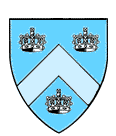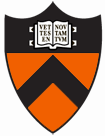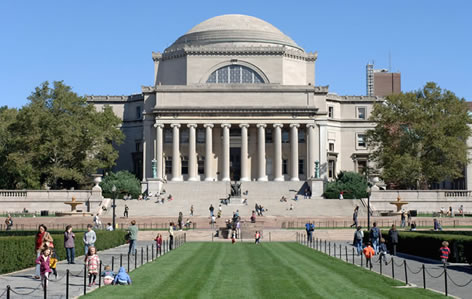Columbia-Princeton Probability Day 2014

Columbia University, April 11, 2014Confirmed Speakers Martin Barlow (U. British Columbia) Thierry Bodineau (Ecole Polytechnique) Steve Lalley (U. Chicago) Subhro Ghosh (Princeton University) Clement Hongler (Columbia University) Jason Miller (MIT) Schedule 9-10: Registration/Breakfast10-11: Barlow 11-12: Bodineau 12-2: Lunch break 2-3: Lalley 3-3:40: Miller 3:40-4:00: Tea break 4-4:40: Ghosh 4:40-5:20: Hongler Practical Information The conference will take place in Columbia University's Northwest Corner Building Room 501, on April 11, 2014. Directions to Columbia's Mornigside Heights Campus. Please register in order to attend the conference. (Registration is free but kindly requested). Local organizers: Julien Dubédat, Ramon van Handel Titles and Abstracts Martin Barlow Quenched and averaged invariance principles for the random conductance model. M.T. Barlow, K. Burdzy, A. Timar. The random conductance model considers a discrete or continuous time random walk on Z^d where the edges e are given random weights mu_e. While the case of i.i.d. mu_e is now essentially solved, many questions remain for stationary ergodic environments. I will discuss the difference between 'quenched' and 'annealed' (or, better, 'averaged') invariance principles in this context. Thierry Bodineau Tagged particle in a deterministic dynamics of hard spheres We consider a tagged particle in a diluted gas of hard spheres. Starting from the hamiltonian dynamics of particles in the Boltzmann-Grad limit, we will show that the tagged particle follows a Brownian motion after an appropriate rescaling. We use the linear Boltzmann equation as an intermediate level of description for one tagged particle in a gas close to global equilibrium. Subrho Ghosh Rigidity phenomena in random point sets and applications In several naturally occurring (infinite) point processes, we show that the number (and other statistical properties) of the points inside a finite domain are determined, almost surely, by the point configuration outside the domain. This curious phenomenon we refer to as "rigidity". We will discuss rigidity phenomena in point processes and their applications. Depending on time, we will talk about applications to stochastic geometry and to random instances of some classical questions in Fourier analysis. Clement Hongler Ising Model: making probabilistic sense of Conformal Field Theory ideas We will consider the 2D Ising model at or near the critical point. The scaling limit of this model is conjecturally described by a minimal model of Conformal Field Theory. We will discuss various aspects of this description, and focus on making rigorous, probabilistic sense of these ideas. Based on joint works with S. Benoist, D. Chelkak, H. Duminil-Copin, K. Izyurov, F. Johansson Viklund, A. Kemppainen, K. Kytölä and S.Smirnov. Steve Lalley Local Limit Theorems  for Random Walks on Hyperbolic Groups A fundamental theorem of Kesten asserts that for any nontrivial random walk on a nonamenable group the n-step return probabilities decay exponentially with n. The precise asymptotic behavior, however, seems to depend on the geometry of the group.  For hyperbolic groups (those whose Cayley graphs have the "thin triangle" property), sharp limit theorems have recently been proved. I will report on  these results and related work concerning  "boundary behavior". Jason Miller Random Surfaces and Quantum Loewner Evolution What is the canonical way to choose a random, discrete, two-dimensional manifold which is homeomorphic to the sphere? One procedure for doing so is to choose uniformly among the set of surfaces which can be generated by gluing together $n$ Euclidean squares along their boundary segments. This is an example of what is called a random planar map and is a model of what is known as pure discrete quantum gravity. The asymptotic behavior of these discrete, random surfaces has been the focus of a large body of literature in both probability and combinatorics. This has culminated with the recent works of Le Gall and Miermont which prove that the $n \to \infty$ distributional limit of these surfaces exists with respect to the Gromov-Hausdorff metric after appropriate rescaling. The limiting random metric space is called the Brownian map. Another canonical way to choose a random, two-dimensional manifold is what is known as Liouville quantum gravity (LQG). This is a theory of continuum quantum gravity introduced by Polyakov to model the time-space trajectory of a string. Its metric when parameterized by isothermal coordinates is formally described by $e^{\gamma h} (dx^2 + dy^2)$ where $h$ is an instance of the continuum Gaussian free field, the standard Gaussian with respect to the Dirichlet inner product. Although $h$ is not a function, Duplantier and Sheffield succeeded in constructing LQG rigorously as a random area measure. LQG for $\gamma=\sqrt{8/3}$ is conjecturally equivalent to the Brownian map and to the limits of other discrete theories of quantum gravity for other values of $\gamma$. In this talk, I will describe a new family of growth processes called quantum Loewner evolution (QLE) which we propose using to endow LQG with a distance function which is isometric to the Brownian map. I will also explain how QLE is related to DLA, the dielectric breakdown model, and SLE. Based on joint works with Scott Sheffield.